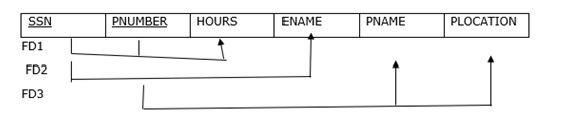# What is functional dependency and transitive dependency (DBMS)?

Let us begin by understanding what a functional dependency is in the database management system (DBMS).

## Functional Dependency

Functional dependency refers to the relation of one attribute of the database to another. With the help of functional dependency, the quality of the data in the database can be maintained.

The symbol for representing functional dependency is -> (arrow).

Example of Functional Dependency

Consider the following table.

Employee NumberNameCitySalary
1bobBangalore25000
2LuckyDelhi40000

The details of the name of the employee, salary and city are obtained by the value of the number of Employee (or id of an employee). So, it can be said that the city, salary and the name attributes are functionally dependent on the attribute Employee Number.

### Example

SSN->ENAME read as SSN functionally dependent on ENAME or SSN
determines ENAME.
PNUMBER->{PNAME,PLOCATION} (PNUMBER determines PNAME and PLOCATION)
{SSN,PNUMBER}->HOURS (SSN and PNUMBER combined determines HOURS)## Transitive Dependency

The transitive dependency is being obtained by using the relation of more than three attributes.

These dependencies are being used to normalize the database in 3NF.

### Example of Transitive Dependency

Consider the following table −

BookBook_AuthorAge_of_Author
ABCHari45
PQRJames60

### The dependencies are as follows −

{Book} -> {Book_Author}
{Book_Author} does not -> {Book}
{Book_Author} -> {Age_of_Author}

Hence, as per the transitivity, the {Book} -> {Age_of_Author}. Therefore, it one knows the book then it must know the age of the Author.

## Problem

A relation R(ABCDEF) and F: {AB->C, C->A, B->DE, ABD-> F}. Find the transitive dependency.

## Solution

AB+=ABCDE => AB is candidate key

C+=CA

B+=BDE

ABD+=ABDFCE =>ABD is not candidate key [since AB is candidate key].

=>key attribute= {A,B} and non-key attributes ={C,D,E}

AB-> C is not a transitive dependency.

C->A is not a transitive dependency.

B->DE is a transitive dependency [Since B is not a candidate key/super key and DE is a non-key attribute}.

ABD->F is not a transitive dependency. [Since, ABD is a super key].